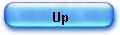THE BUGS in HP 35 early ROM

"exp(ln (2.02))=2" bug fully elucidated

My series of articles about the HP 35 ROM reverse engineering was almost finished, when Peter MONTA published a dump of the early ROM with bugs (Feb 2006). He kindly gave me a raw disassembly listing.

At that time (end of January 2006), I was about myself to dismantle a version 2 machine (with bugs) and to build and plug a "ROM dumping" device to study the code (quite a hard job, anyway). I decided to leave all other projects, and get back to the ROM listing.

Remember the context: HP had already sold 25 000 HP 35 when a problem of accuracy was discovered in several cases. HP offers a replacement and around 5000 machines were returned to have their 3 ROMs exchanged.

I still own an old working “buggy” ROM version 2 machine.

Are concerned (to my knowledge): version 1 (red dot + label HEWLETT PACKARD instead of HEWLETT PACKARD 35 and a few version 2 models (no red dot – I own also one of this kind).

The most visible bug is the exponential one: exp (ln (2.02)) = 2 instead of 2.02!

I have worked on the “new old” listing only a few hours, and there are more than 100 test cases to pass to see exactly what’s going on, comparing every trace with its new ROM counterpart. But here are partial results. Take this, please, as a blog rather than as a formal paper!

1) Partial results

I started to put “labels” in the disassembly, identify the routines I know and track differences.

Many differences are not changes but just routine order moves or “jsb” and “goto” referring to different octal addresses but to same labels:

- in ROM 0.

Many changes. Cochran re-wrote all the “den” and “eex” digit entry routines, mainly to fix the “CHS” bug (strange behaviour when used during exponent entry) and he made changes here and there, in “of” routine for example.

Routines “dsp1” and “fst” are the same.

- ROM 1.

2 changes at address 01117 and 01120 (part of trigonometric routine) regarding rounding off hence accuracy.

- ROM

8 changes at addresses: 02027, 02105, 02122, 02123, 02153, 02221, 02223, 02230, 02231.

All are related to Cordic "ln" and "exp" algorithms and more precisely to accuracy errors due to accumulating very small errors in elementary operations and/or in prescaling operations.

2) Bug hunting party: exp(ln (2.02)) = 2

Re-reading yesterday (12 March) the Meggitt's paper (page 223), I suddenly understood (using my brain and a few cups of coffee, instead of the simulator!) who was the culprit.

I took a HP35 new Rom listing (no bugs exp(ln(2.02))= 2.02), replaced only two lines of code with 2 “no operation” instructions at address 02122 and 02123 and voilà, my new machine HP35 version X, just displayed what it must not:   exp(ln(2.02))=2.

New ROM

678   L02122:  .11...11..                               6 -> p
679   L02123:  1.1111..1.                              0 -> a[wp]

New ROM patched

678   L02122:  ..........                               no operation
679   L02123:  ..........                               no operation

The complete explanation would take a complete article by itself but for now, I will quote Meggitt (35 years later!): “The number that is in A at the end of the process is the required answer and it is necessary to find how any digits of it are accurate. Inaccuracies occur in the pseudo multiplication process, due to the dropping of figures when the pseudo multiplicand is updated”.

In the case of exp(ln(2.02), at the end of part 1, the following constants have been loaded from ROM: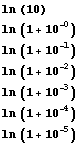and the pseudo quotients have been computed by repetitive subtractions.

But there is remainder after the subtractions cycle is completed (the part2 of the calculation uses the routine “eca”, to perform pseudo multiplications on the number in register A) and all the digits of this remainder are not significant.

Here is the result in a new ROM patched:

02174:             return
A=00487311935000  B=59900107030000  C=00999999500000
D=00000000000000  M=00000000000000  P=5  S=0.2....7.9..

02122:             no operation
A=00487311935000  B=59900107030000  C=00999999500000
D=00000000000000  M=00000000000000  P=5  S=0.2....7.9..

02123:             no operation
A=00487311935000  B=59900107030000  C=00999999500000
D=00000000000000  M=00000000000000  P=5  S=0.2....7.9..

And in a factory new one:

02174:             return
A=00487311935000  B=59900107030000  C=00999999500000
D=00000000000000  M=00000000000000  P=5  S=0.2....7.9..

02122:             6 -> p
A=00487311935000  B=59900107030000  C=00999999500000
D=00000000000000  M=00000000000000  P=6  S=0.2....7.9..

02123:             0 -> a[wp]
A=00487310000000  B=59900107030000  C=00999999500000
D=00000000000000  M=00000000000000  P=6  S=0.2....7.9..

The correction was simple: deleting the 7 right most digits of the remainder.

More on this later, I promise.
Meanwhile, here is a version X ROM simulator and the ROM listing with its 2 instruction patch.

Monday, 13 March 2006.

3) Investigation developments:  more about the exp(ln(2.02)) bug.

I’ve done a lot of calculations last night to close this chapter, before flying south, for a few days off.
I enclose an Excel sheet that computes correctly “exp(ln(2.02))” = 2.02 with its own level of accuracy.

Part 1 of the algorithm leads to a remainder r = 0.00000487308930222163.

It helps testing the sensibility of the final result to variations of the remainder, when a few right most digits are chopped off.

The result varies but never goes from 2.02 to 2, knowing that this behavioural degeneracy happens for certain values only.

The cure too is puzzling too. The designer reduced numerical precision of the input (the remainder) to increase precision of the output!

It seemed to me, yesterday, that I found the murderer but not yet the type of poison he used.

Now, I got it.

As I have said previously, I made me a special ROM with 2 missing instructions at address 02122 and 02123.

I replaced the two lines by 2 “no operation” instructions. As forecasted the simulator displayed a wrong result: exp(ln(2.02)) = 2.

Deleting the 7 right most digits of the remainder solved the problem. Quite astonishing!

In fact, the solution is a little more subtle.

The second part of the HP 35 exponential algorithm has flaw in it, but to be more precise, for certain values; there is an eccentricity in its behaviour.

Please read again, my explanations in the article “HP 35 ex Algorithm” and particularly regarding the fact that the part 2 ("pseudo multiplications”) start with the remainder + 1 (r + 1).

To have “only shift and add operations, a simple arrangement is made: we’ll add 1 at the leftmost rank of the mantissa a[p] (byte 12), after each pseudo multiplication.”

This works well most of the time, but in the old ROM, combined with the 7 right most on significant digits that were left there: successive additions and right shifts lead -possibly- to some idiosyncrasy.

I enclose a trace file (Excel) of the algorithm's second part on the 2 ROMS, at left the buggy, at right the new ROM.

The bug creeps during successive pseudo multiplications and at the end of q3 processing (marked in red in the trace file):

 A=39000000005108  B=38991008996112  C=00010703000000 A=38999999985563  B=38991008976587  C=00010703000000 2207: 11111...1. a + 1 -> a[p] 02207: 11111...1. a + 1 -> a[p] A=30000000005108  B=38991008996112   C=00010703000000 A=39999999985563  B=38991008976587  C=00010703000000

The instruction a + 1 -> a[p] (p=12) overflows, in position 12, there is no room to keep the information, and the result fall drastically form 39000000005108 to 30000000005108.

A few steps further, the result is  A=02000000000010 for the buggy ROM and  A=02019999999970  for the corrected one. After normalization the display shows 2 in the first case and 2.02 in the second.

Wednesday, 15 March 2006

4) Exp(ln(2.02)) bug : the final report.

To fully understand the algorithm used in HP35 for ex function please refer to my dedicated article on this site or to the J.E. MEGGITT’s paper (page 220).
Meggitt insisted that it is the “reverse process of what would be done in finding” the logarithm for positive numbers.

It's a two part algorithm, first the pseudo quotients qj “are found by a pseudo division and in the second the exponential is evaluated by a pseudo multiplication”.

David Cochran followed closely Meggitt and used the same constants in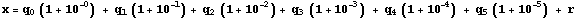as for the logarithm process.

In part one, the argument x is broken in small quantities and the pseudo quotients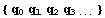are computed by repetitive subtractions.

Next in part two the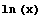process will be reversed and the exponential will be computed by pseudo multiplications: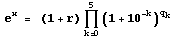.

As we know now, the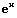algorithm must use the same routine in ROM as for, the same constants, and the same accuracy level to assure symmetry.

I will conduct my demonstration in 2 steps.

First, I will explain the symptom: why in the process of the algorithm (part 2), the calculation seems to fall in an “air pocket”!

Next, I will explain why, “This situation involves only the use of certain numbers” (as explained in the HP 35 Errata sheet).

4.1) The Pseudo multiplication flaw.

For the argument x=2.02, the part one of the algorithm gives “599001” for result (pseudo quotients).

It is the number of times each constant could be subtracted (5 times ln(1.00001), 9 times ln(1.0001), 9 times ln(1.001) and 1 time ln(2)).

The remainder after the subtractions is 0.00000487311935.

Part 2 of the algorithm is a pseudo multiplication process (in fact additions and right shifts) : each constant is pseudo multiplied qj times (see full trace for 2.02 on the buggy ROM).

The calculation starts with the remainder + 1 (r + 1) and to have “only shift and add” operations, 1 is added at the leftmost rank of the mantissa a[p] (digit 12), after each pseudo multiplication.

The problem occurs at the third step while processing q3=9: the table below shows the sequence.

 q5=5, SR=5 599001 0 50 487 311 935 000 q5= 5 1 51 487 316 808 119 q4= 9 2 52 487 331 681 287 q3= 9 3 53 487 356 554 603 4 54 487 391 428 168 5 55 487 436 302 082 q4=9, SR=4 0 40 548 798 504 571 1 41 548 953 384 421 2 42 549 208 279 759 3 43 549 563 200 586 4 44 550 018 156 906 5 45 550 573 158 721 6 46 551 228 216 036 7 47 551 983 338 857 8 48 552 838 537 190 9 49 552 838 537 190 q3=9, SR=3 0 30 956 239 137 572 1 31 956 239 137 572 2 32 958 195 376 709 3 33 961 153 572 085 4 34 965 114 725 657 5 35 976 049 920 222 6 36 983 025 970 142 7 37 983 025 970 142 8 38 991 008 996 112 9 30 000 000 005 108 20 000 000 000 510

Digit 13 of register A holds the number of SR (5, 4, 3 etc) according to the constant (5 SR for ln(1.00001) etc…).

Digit 12 is incremented after each pseudo multiplication, as explained above.

In a normal process (for one qj) digit 12 will remain < 10 ; and in fact it is almost the case. But there, the mathematical analysis of the implementation is weak.

With the argument x = 2.02 at the 8th step of the process for q3, we have A= 38 991 008 996 112!

At the 9th step, register A can’t place the carry and overflows A=30 000 000 005 108 leading a few steps later to the wrong result 2.0.

What went wrong?

As one can see, the first q5 process has no effect on the 3 left most computed digits (pattern 487).

-  q4 process takes the result q5=5 to form the 5XX pattern (548, 549, 550, 551, 552),

-     q5 process takes the result q4=9 to start the  9XX pattern (956, 958, 961, 965, 976, 983, 991).

So it happens that the algorithm, as is, cannot stand pseudo quotient result as “599001” where two 9 are in 4th and 3thd position.

But, It is easy to see (in the full trace) that the overflow of A register is due to 2 concurrent causes:
1) the unexpected pattern of the pseudo quotient (“599001”) and
2) the presence of non significant digits in the remainder result
: take 0.0000048731 instead of 0.00000487311935 and you'll get rid of the overflow. 4 digits that cost a lot!

That’s the solution D. Cochran took in the new Rom inserting 2 instructions at the end of the part1, to cut off the 7 rightmost digits in A:

02122: .11...11..                     6 -> p
02123: 1.1111..1.                    0 -> a[wp]

It’s enough to correct this particular flaw, though the designer did other corrections regarding accuracy. To prove this, I simply replaced -in the new Rom- these 2 lines by 2 "no operation" instructions, and the wrong result reappears.

4.2) The so called “idiosyncrasy”

The HP35 errata sheet says “The idiosyncrasy is in the ey function, not in the logarithmic function.”

Correct. But what it is?

It came very soon in my mind that the pseudo quotient result calculated by the algorithm was strange “599001” since:

ln(2.02) = ln( 2 x 1.01) = ln(2) + ln(1.01), so that we can -in theory- exactly subtract ln(2) and ln(1.01) and have a theoretical pseudo quotient result of ”000101”.

Now, what's going on is clear in my mind (I hope in my explanations too).

To the number 0.7030975114, the algorithm first subtracted ln(2) stored as 0.693147180553, the result is r=0.009950330847 (A=00009950330847)

Next, the process tried to subtract ln(1.1) and failed normally.

But at the next step, we meet the idiosyncrasy.
The algorithm loads from ROM, ln(1.01)  = 0.00995033085090  and try to make the subtraction

r = 0.009950330847 (previous remainder)
-    0.00995033085093

but alas it is impossible due to difference of precision: r < 0.00995033085093 ; the constants in ROM are stored left aligned with a maximum of precision whereas ln(2.02) =  0.7030975114 has been computed previously hence normalized as C=07030975114999.

The algorithm fails then for ln(1.01) and subtracts a maximum of 9 times ln(1.001), 9 times ln(1.0001) and 5 times ln(1.00001).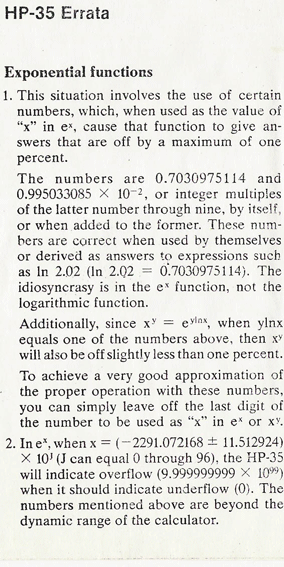The pseudo quotient is “599001” where it should be “000101” and this unexpected pattern causes a register overflow.

The text of the errata sheet sent to the early 25000 owners refers to another number 0.995033085 x 10E-2.

Obviously it is the number ln(1.01) !

For each attempt to subtract to the argument x the number ln(2) and ln(1.01) possibly multiplied by an integer value, the algorithm will flaw:

–  type .995033085 EEX CHS 2 and ex, the result will be “1.” Instead of 1.01, on a buggy machine,
– type .0199006617 ex and the result will be 1.01 instead of 1.02.

Note that's this behavior is rather touchy (it depends on a couple of digits at the 12th or 13th rank), e.g. if you type –on a buggy machine- ex(ln(1.01)) instead of  the sequence .995033085 EEX CHS 2 and ex, then the result is good ; or if you type the sequence " .995033085 EEX CHS 2 ENTER 5 * " and then ex then the result is wrong : 1.04...

There are many other comments to make regarding the differences between the old buggy HP 35 ROM and the new one.

I will publish them as soon as my understanding is assured.
Feel free, if you are interested to get in touch with me.

The original errata sheet (photo J.Laporte)

During my spring vacation, I point was puzzling me: was the correction a final one of just a temporary fix?

One fact is sure, if you look, as I did, in the HP45 ROM listing, you’ll find the same 2 line correction:

686   L02122:  .11...11..                                    6 -> p
687   L02123:  1.1111..1.                                   0 -> a[wp]
688   L02124:  11.1..11..                                   13 -> p
689   L02125:  1...1.111.                                    b exchange c[w]
690   L02126:  111.1.111.                                   a exchange c[w]
691   L02127:  .11..11...                                    load constant 6
692   L02130:  1...111.11                                   go to exp23

and you can find them in the HP25 Rom :

.../...
1628 L03133: ..11.11..1     jsb pqo21
1629 L03134: ..11.11..1     jsb pqo21
1630 L03135: 1.111111..     p <- 6
1631 L03136: .......11.       0 -> a[wp]
1632 L03137: 1.1.1111..     p <- 13
1633 L03140: ..11111.1.     b exchange c[w ]
1634 L03141: ..1..11.1.     a exchange c[w ]
.../...

and also in the HP-65 Rom.

1872 L07117: 1..11.11.1 jsb pqo21
1873 L07120: 1..11.11.1 jsb pqo21
1874 L07121: 1..11.11.1 jsb pqo21
1875 L07122: .11...11.. 6 -> p
1876 L07123: 1.1111..1. 0 -> a[wp]
1877 L07124: 11.1..11.. 13 -> p
1878 L07125: 1...1.111. b exchange c[w]
1879 L07126: 111.1.111. a exchange c[w]

Jacques Laporte,